Browse by Topic
Related Topics

# Mathematica Q&A: Combining and Annotating Plots

Got questions about Mathematica? The Wolfram Blog has answers! We’ll regularly answer selected questions from users around the web. You can submit your question directly to the Q&A Team using this form.

Today’s question is from Herbert, a reader of this blog:

How can I plot a function like sin(x) together with a relation like x = π?

You can plot two or more functions together by giving a list as the first argument of a function like Plot: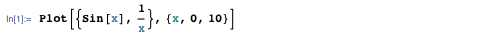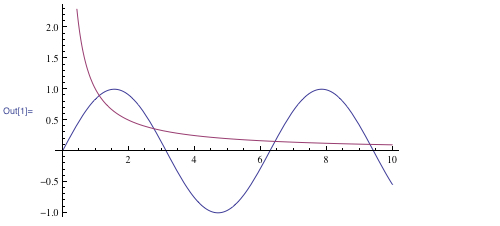But a relation like x = π is not a function, since it doesn’t define a single function value for each x.

There are a few different ways to combine a regular plot of a function with a visualization of a relation.

The particular relation x = π is a single vertical line. Any arrangement of horizontal and vertical lines can be produced using the GridLines option: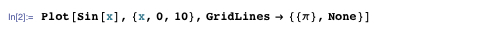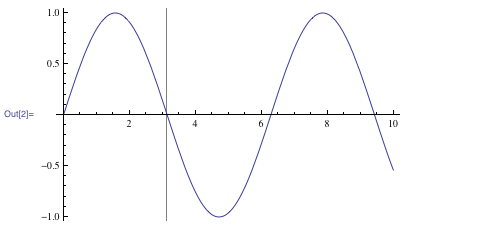The setting GridLines → {xlist, ylist} draws grid lines at the specified lists of points on the x– and y-axes. You can also use GridLinesStyle to specify colors and styles. Here’s a version with multiple dashed grid lines: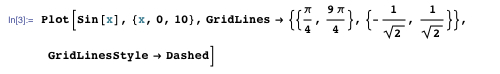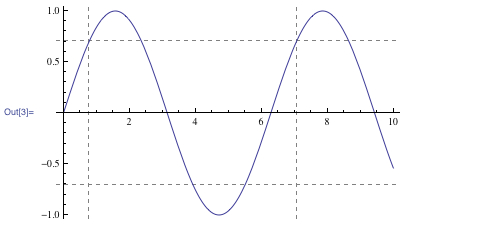Another way to get the vertical line x = π is to explicitly tell Plot to draw the line after it finishes plotting. You do this with the Epilog option: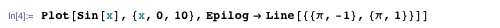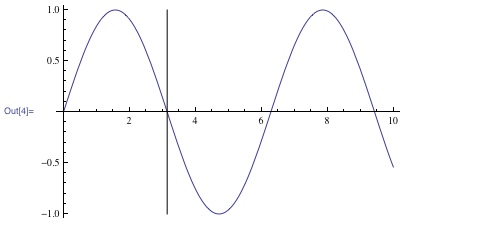The Epilog option can include any combination of Mathematica‘s graphics directives, such as colors and dashing: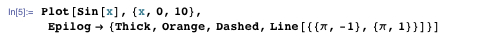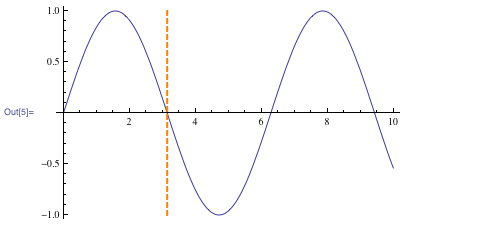Instead of manually drawing the line representing x = π, you can use ContourPlot to visualize the relation: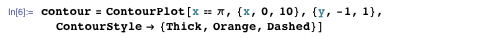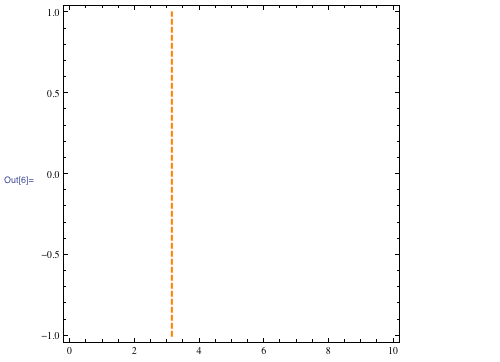Using the function Show, you can combine the contour plot with a regular plot of sin(x):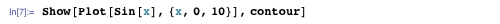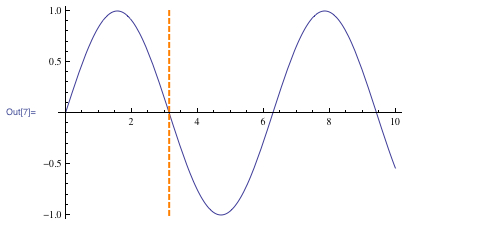(Note that Show uses the plot size and axes of its first argument—in this case, the regular plot of sin(x).)

The advantage of ContourPlot is that you can readily visualize more complicated relations than x = π. Here’s the relation sin(x) = sin(y):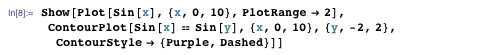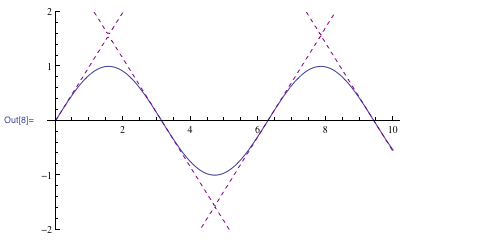You can use Show to combine the graphics produced by any of Mathematica‘s graphics functions. For example, RegionPlot can be used to visualize regions satisfying inequalities. Here’s a plot highlighting the region satisfying both sin(x) < y and x < π/2: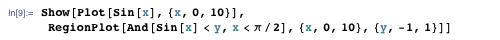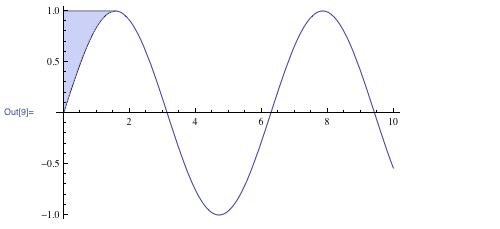Between Show, options like GridLines and Epilog, and all of Mathematica‘s built-in graphics functions, you have a lot of flexibility when creating custom visualizations.